## Explain This?! - Model Interpretability

Interpretability also popularly known as human-interpretable interpretations (HII) of a machine learning model is the extent to which a human (including non-experts in machine learning) can understand the choices taken by models in their decision-making process.

A machine learning model by itself consists of an algorithm which tries to learn latent patterns and relationships from data without hard-coding fixed rules. This makes explaining how a model works in a business context often difficult to do. This has driven a recent surge of interest into the idea of being able to interpret models to explain the how and why models make the predictions they do.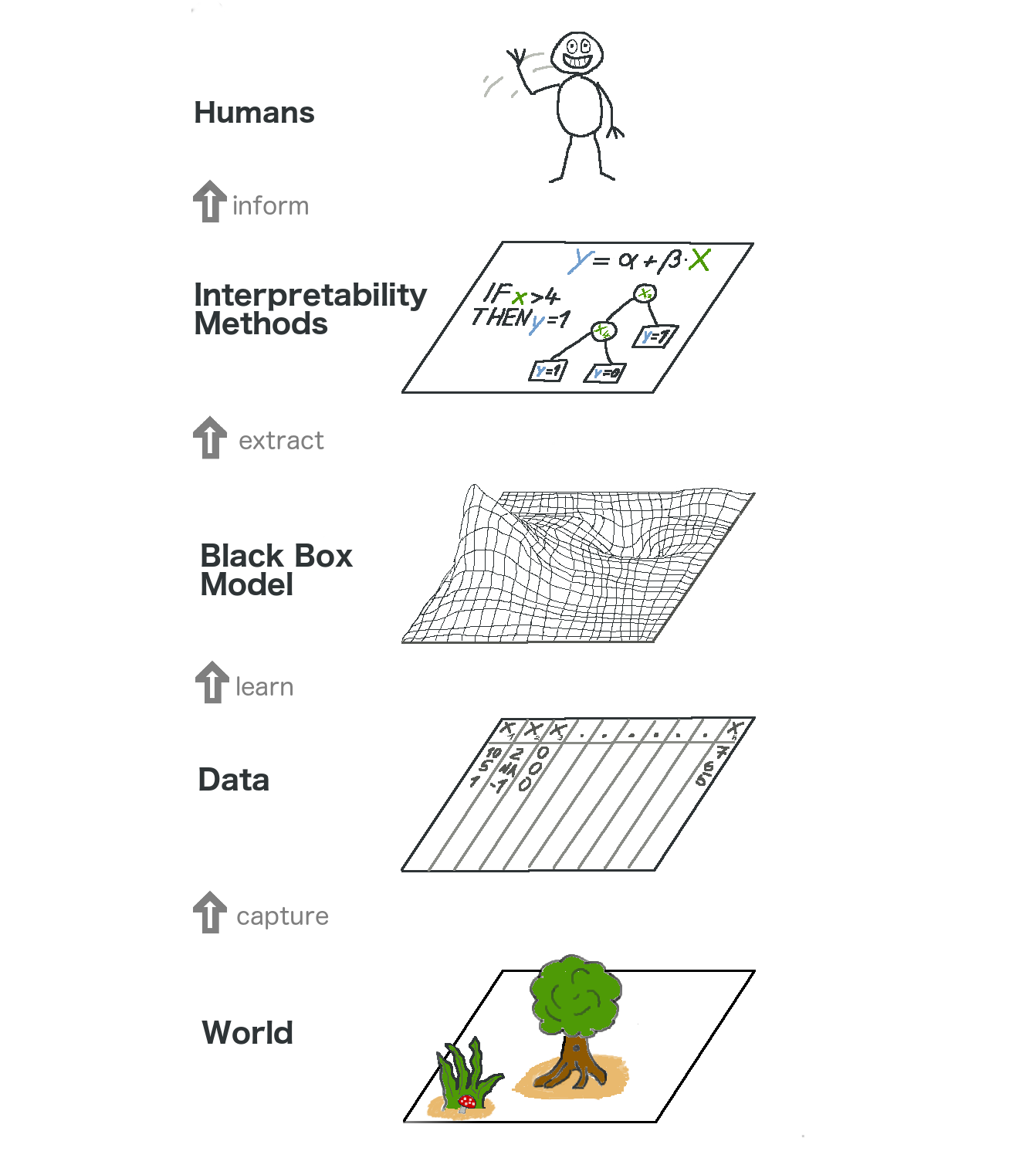Interpretable ML - The Big Picture

### Key Concepts

##### Intrinsic vs. Posthoc

Certain models, such as linear or tree-based models, have been designed in such a way that they are intrinsically interpretable by nature. For example, linear models are monotone and the dependant variable scales linearly with a predictor variable; this means the weights, or coefficients, that controls this behaviour can be understood simply. In the same manner, simple tree-based methods have features and thresholds which make human sense.

By comparison, post-hoc interpretability refers to techniques that are applied after training to models that can be considered 'black box' where the internals are hard to decipher. An example of a post-hoc method would be permutation feature importance, where a trained model is applied to a dataset when the input data has been shuffled, and the importance of the feature is assessed by the change of the prediction from what was expected.

##### Model Specific vs. Model Agnostic

Interpretability techniques that are restricted to the model it is be applied on is considered model specific. Therefore, intrinsic techniques are by definition model specific. Model agnostic techniques are those that can be applied on any trained model, hence are often post-hoc.

##### Local vs. Global

If we are able to explain an entire model's behaviour, i.e. describe how a model will perform against the whole feature space, then we call such a technique global. In contrast, if we can explain why a specific prediction was made, then the technique is considered to have local interpretability.

### Techniques

In this post, I will describe some commonly used post-hoc, model-agnostic techniques. I will present high level theory and give example applications using models trained on MNIST Digits and the Boston House Prices datasets.

The following code snippet shows how I quickly and naively build a few regression and classification models.

```    from sklearn import datasets
import pandas as pd

boston_X = pd.DataFrame(boston.data, columns=boston.feature_names)
boston_y = pd.DataFrame(boston.target, columns=['prices'])

iris_X = pd.DataFrame(iris.data, columns=iris.feature_names)
iris_y = pd.DataFrame(iris.target, columns=['flower'])

from sklearn.linear_model import LinearRegression, LogisticRegression
from sklearn.tree import DecisionTreeClassifier, DecisionTreeRegressor
from sklearn.ensemble import RandomForestClassifier, RandomForestRegressor
from xgboost.sklearn import XGBClassifier, XGBRegressor

from sklearn.model_selection import train_test_split

from sklearn.metrics import accuracy_score, r2_score

def train_model(X, y, model, metric):
X_train, X_test, y_train, y_test = train_test_split(X, y, test_size=0.8, random_state=42)

model.fit(X_train.values, y_train.values)
y_test_hat = model.predict(X_test.values)
score = metric(y_test, y_test_hat)
print(f'Model {model.__class__.__name__} achieved a {score} score.')

return model

lin_reg = train_model(boston_X, boston_y, LinearRegression(), r2_score)
dec_tree_reg = train_model(boston_X, boston_y, DecisionTreeRegressor(), r2_score)
ran_for_reg = train_model(boston_X, boston_y, RandomForestRegressor(), r2_score)
xgb_reg = train_model(boston_X, boston_y, XGBRegressor(), r2_score)

log_reg_clf = train_model(iris_X, iris_y, LogisticRegression(), accuracy_score)
dec_tree_clf = train_model(iris_X, iris_y, DecisionTreeClassifier(), accuracy_score)
ran_for_clf = train_model(iris_X, iris_y, RandomForestClassifier(), accuracy_score)
xgb_clf = train_model(iris_X, iris_y, XGBClassifier(), accuracy_score)
```

### Feature Importance

Feature importance is a generic term for the degree to which a predictive model relies on a feature. To understand if input features are important, and how significantly they are so, can give incredible insight into what are the driving factors in a predictive model.

The intuition behind most techniques is that you can determine how much a model relies on a particular feature by making slight changes to the values of that feature in the dataset and comparing the predictions with the baseline. You can say that there is high reliance on that feature if small changes in the feature have a large effect on the predictions. In contrast, a feature is not important to a model if you can change values in that feature with the predictions remaining mostly unchanged.

#### ELI5

This package determines the importance of a feature by attempting to make a prediction without that feature present and comparing the new score with the original. It avoids retraining any model without the feature present by replacing all data within that feature with random noise. In this case, the random noise is generating by randomly shuffling all data within that feature column (hence they are selecting data with the same distribution) and then comparing predictions.

##### Global
```    import eli5
from eli5.sklearn import PermutationImportance
perm = PermutationImportance(lin_reg).fit(boston_X, boston_y)
eli5.show_weights(perm, feature_names=boston_X.columns.values)

perm = PermutationImportance(log_reg_clf).fit(iris_X, iris_y)
eli5.show_weights(perm, feature_names=iris_X.columns.values)
```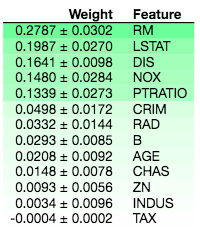ELI5 feature importance on Boston House Price dataset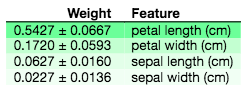ELI5 feature importance on Iris dataset

The output on the top shows the feature importance of a LinearRegression when applied to the Boston House Price dataset. It shows that the RM and LSTAT features are the most important. The output on the bottom then shows that petal length and petal width are the most important predictors for the LogisticRegression classifier. For more information on how to interpret the weight figure, read here.

#### SHAP

This package applies the Shapley value concept from game theory to machine learning. The Shapley value of a feature (player) is the average marginal contribution towards the prediction (payout) across all feature values (coalitions). The marginal contribution is based on the difference from the average prediction made. Edward Ma and Christoph Molnar explain this idea with some fantastic diagrams in a very approachable manor.

##### Global

To visualise feature importance using Shapley values as your metric, you initialise an 'Explainer' class with the model you are inspecting, along with the input data you trained the model with. Then you calculate the Shapley values and feed this array into one of the various plotting methods available from the SHAP library.

In the plot below, we show the average absolute value of all of the Shapley values by input feature; this output can be interpreted to say that the RM, DIS, and LSTAT features are the most impactful in influencing predictions across the whole set of input data.

```    explainer = shap.LinearExplainer(lin_reg, boston_X)
shap_values = explainer.shap_values(boston_X)
shap.summary_plot(shap_values, boston_X, plot_type='bar')
```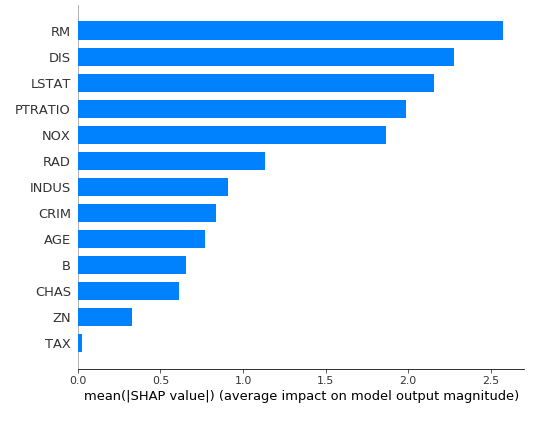Global SHAP feature importance on Boston House Price dataset

To look deeper into feature importance, you can visualise the distribution of individual Shapley values by input feature. This can be useful in determining if there are subsets of the input data where there are skewed values from different features which could be significantly affecting prediction values.

For example, in the following plot you can see that the CRIM feature will have a large negative effect on the predicted house prices when the feature value is low; but when the feature value is high, it will have no effect on the predicted values.

```    shap.summary_plot(shap_values, boston_X)
```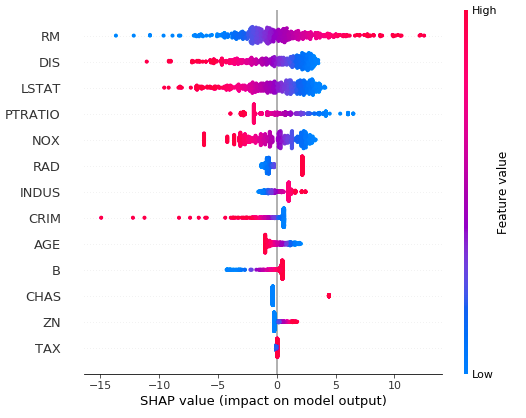Global SHAP feature importance on Boston House Price dataset

##### Local
```    explainer = shap.LinearExplainer(lin_reg, boston_X)
shap_values = explainer.shap_values(boston_X)

# visualize the first prediction's explanation (use matplotlib=True to avoid Javascript)
shap.force_plot(explainer.expected_value, shap_values[0,:], boston_X.iloc[0,:])
```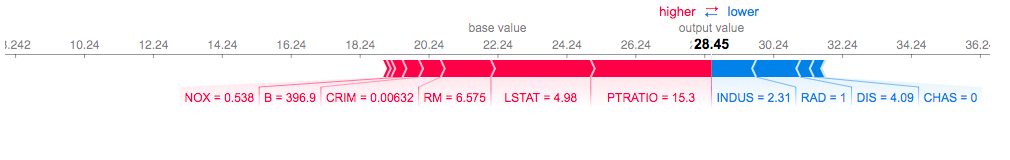Local SHAP feature importance on Boston House Price dataset

You can interpret this output by knowing that the base value refers to the expected output of the model given the training set the model is built on. The arrows and associated features then show which features of this particular sample has contributed to a shift away from the average output. In this example, the LSTAT and PTRATIO has had the largest effect in predicting the value of 28.45.

### Partial Dependency Plots

The partial dependence plot (PDP or PD plot for short) shows the marginal effect one or two features have on the predicted outcome of a machine learning model (J. H. Friedman 200127). A partial dependence plot can show whether the relationship between the target and a feature is linear, monotonous or more complex. For example, when applied to a linear regression model, partial dependence plots always show a linear relationship. The idea of PD plots is similar to plotting the gradient, or value of the partial derivative, of one or two input features.

The reason you can only can only effectively plot dependency for a maximum of two input variables is an artefact of our 3 dimensional world and how visualisations like these are shown on a 2-dimensional screen; mathematically speaking, the analysis would work for any number of dimensions, but interpretation works best at most 2 or 3 dimensions.

#### PDPBox

One Python package you can use to visualise these marginal effects is called PDPBox. You can plot a partial dependence graph with

```    from matplotlib import pyplot as plt
from pdpbox import pdp, get_dataset, info_plots

feature = 'LSTAT'

# Create the data that we will plot
pdp_goals = pdp.pdp_isolate(
model=ran_for_reg,
dataset=boston_X,
model_features=boston_X.columns.values,
feature=feature)

# plot it
pdp.pdp_plot(pdp_goals, feature)
plt.show()
```

and the output will be like the graph below. The X axis shows the feature values you are plotting against, the y axis is the difference in predicted values when compared to the left most X value. The light blue band shows the confidence level in this analysis. With the plot below, you can say that an increasing LSTAT value (the % of the population that is deemed 'lower status'), has a negative effect on house prices until a value of 20 where it has very little effect.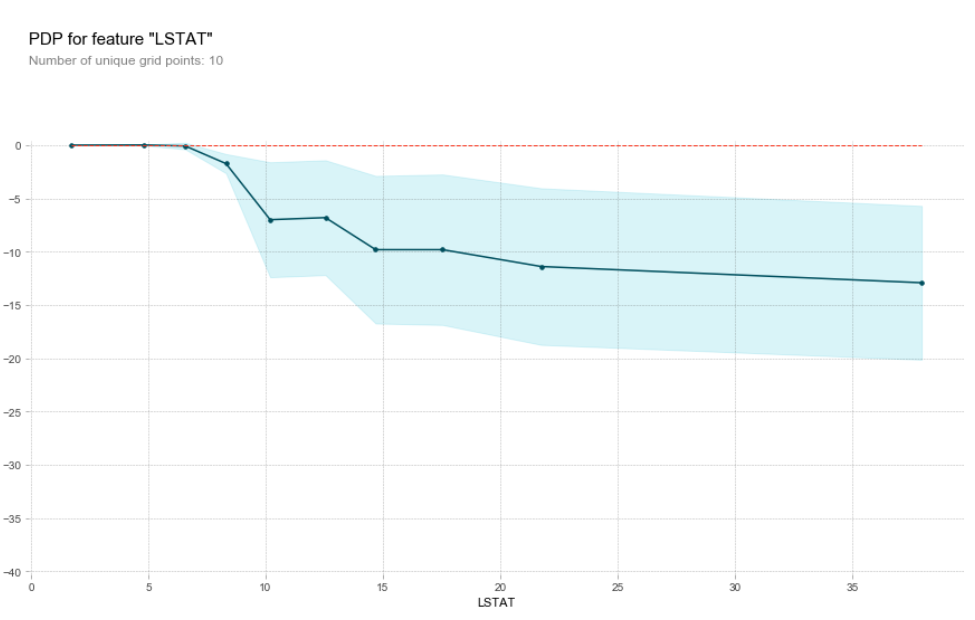Partial dependacy plot for LSTAT feature

The graphs below show the effects of two more variables. RM, the number of rooms in a house has a positive effect on house prices after a value of 6, and CHAS, a dummy variable, has no effect on house prices.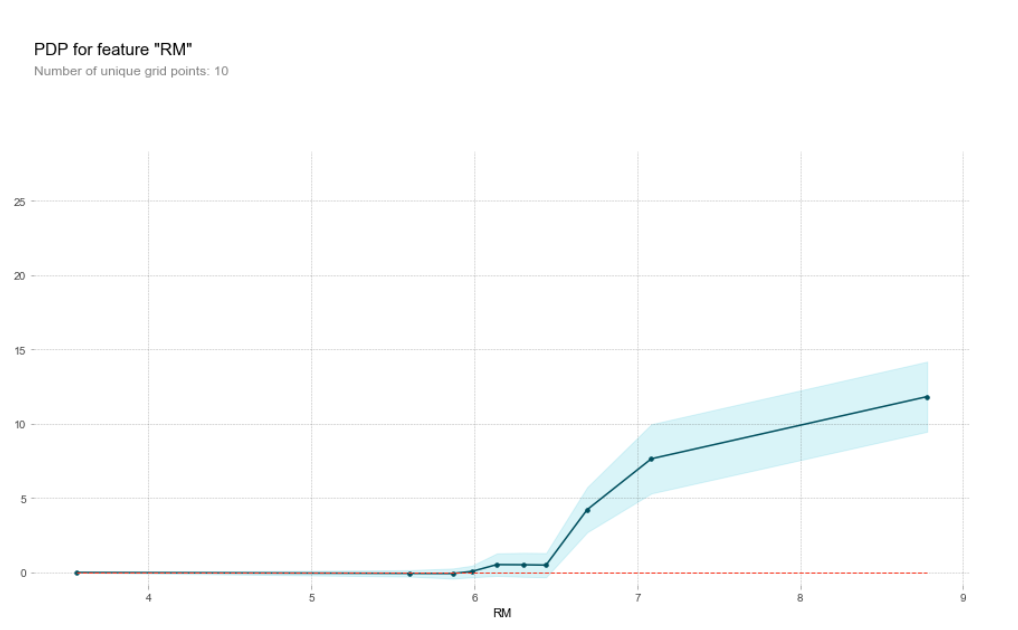Partial dependacy plot for number of rooms feature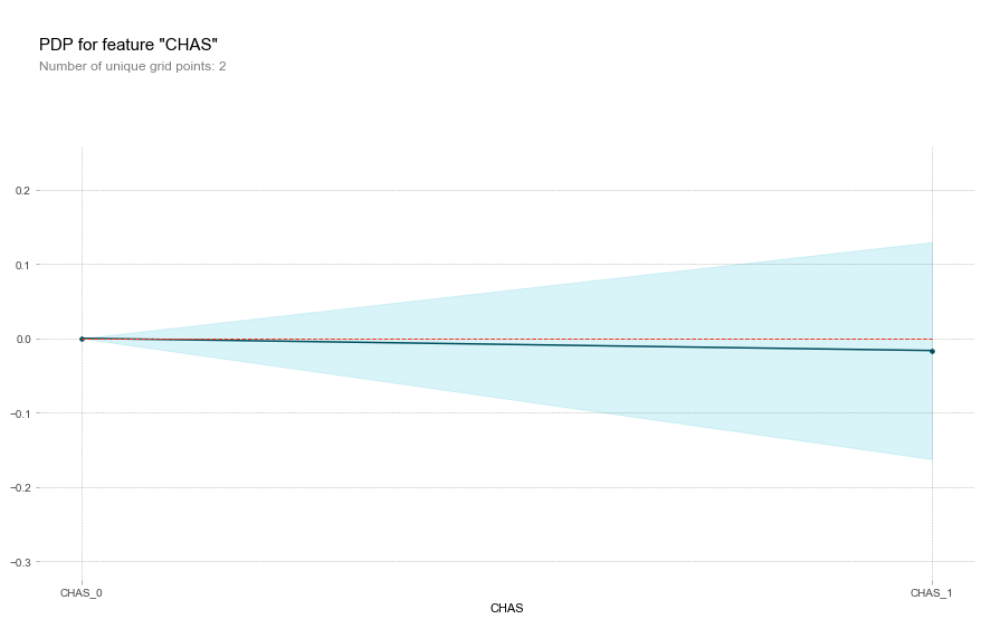Partial dependacy plot for CHAS feature

You can also use PDPBox to visualise the effect of two variables on the predicted value with

```    from matplotlib import pyplot as plt
from pdpbox import pdp, get_dataset, info_plots

features = ['LSTAT', 'RM']

# Create the data that we will plot
pdp_goals = pdp.pdp_interact(
model=ran_for_reg,
dataset=boston_X,
model_features=boston_X.columns.values,
features=features)

# # plot it
pdp.pdp_interact_plot(pdp_goals, features, plot_type='grid')
plt.show()
```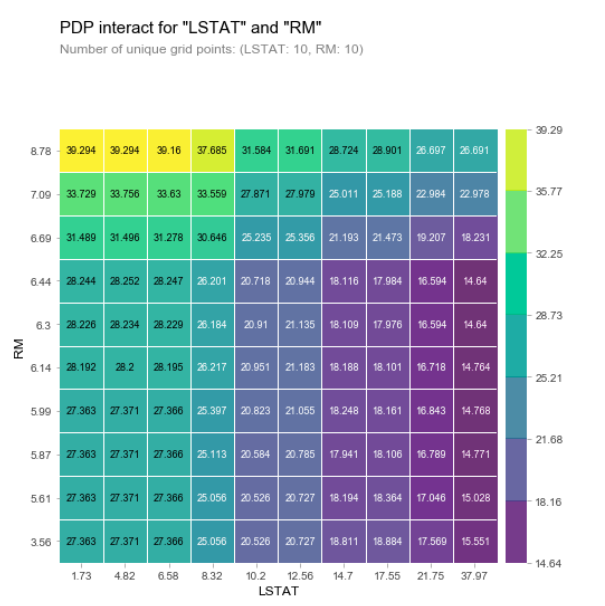Partial dependacy plot interaction plot

The lighter indicates a strong positive effect, and the darker colour indicates the opposite. This plot shows that a higher value of RM and a lower value of LSTAT has a greater positive effect on house prices.

#### Skater

You can find other packages that can do similar analysis to determine partial dependence. Skater, built by Oracle, is another example which has an equally simple API and some beautiful and clear visualisations. Here are the equivalent partial dependence plots using the implementation by Skater. The 1-feature plots are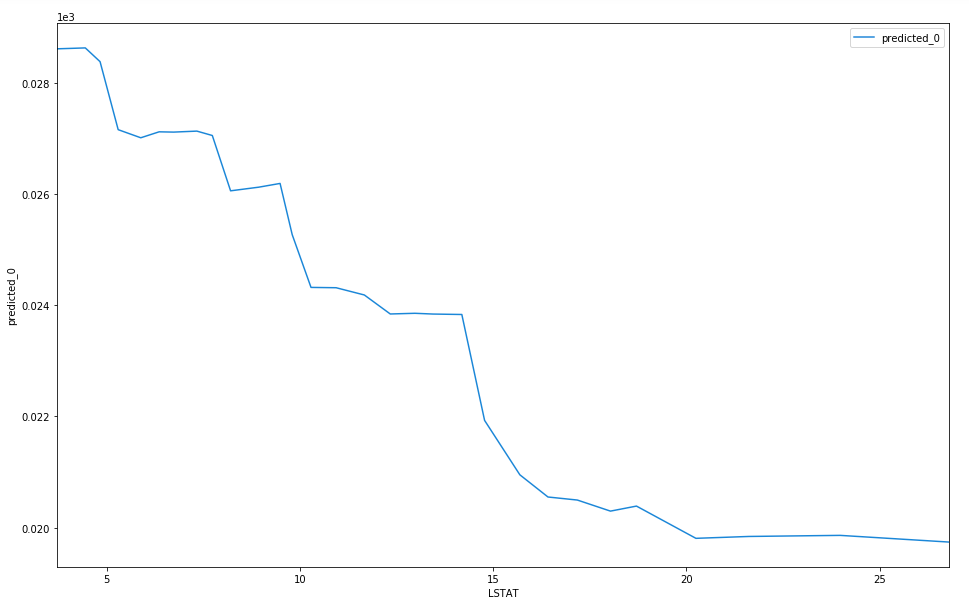Skater partial dependacy plot for LSTAT feature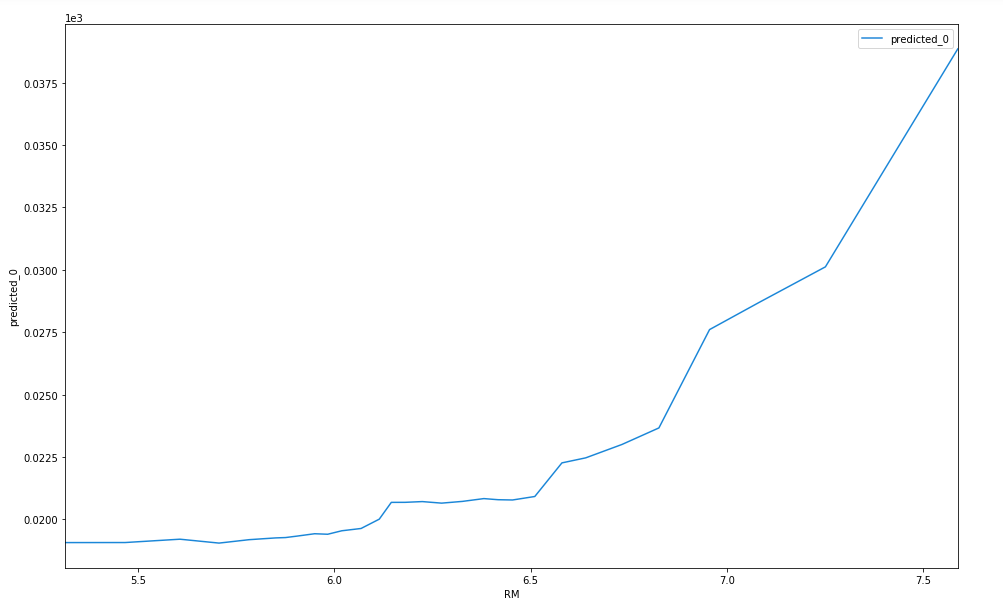Skater partial dependacy plot for number of rooms feature

This can be generation using code like

```    from skater.core.explanations import Interpretation
from skater.model import InMemoryModel
from matplotlib import pyplot as plt

f, ax = plt.subplots(1, 1, figsize = (26, 18))

interpreter = Interpretation(boston_X, feature_names=boston_X.columns.values)
pyint_model = InMemoryModel(ran_for_reg.predict, examples=boston_X)

features = ['LSTAT', 'RM']

interpreter.partial_dependence.plot_partial_dependence(
features,
pyint_model
)
```

and the 2-feature plot is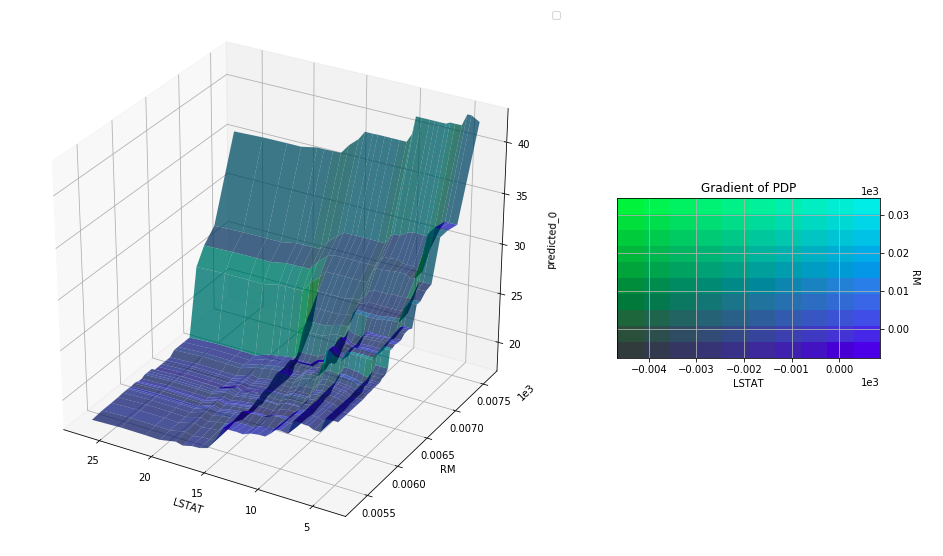Dual partial dependacy plot for LSTAT and RM features

This can be generated by almost identical code through changing

```    interpreter.partial_dependence.plot_partial_dependence(
features,
pyint_model
)
```

to

```    interpreter.partial_dependence.plot_partial_dependence(
[features], # max length 2
pyint_model
)
```

The interpretation of the features and this model through both packages are the same.

### Surrogate Models

The idea of surrogate models is to use an interpretable model that is trained to approximate the predictions of a black box model. If the approximation is close enough, you can draw conclusions about how the black box model makes decisions by using the intrinsic interpretability of the surrogate model.

#### Skater - Global

We can use the tree_surrogate method from the Skater package to train a Decision Tree on the outputs of the base model. Here I train a tree based on the outputs of the XGBoost classifier built on the Iris flower dataset. I can generate a very good decision tree as an approximator with it achieving a 0.993 f-1 score on the XGBoost output with the following code.

```    interpreter = Interpretation(iris_X, feature_names=iris_X.columns.values)
pyint_model = InMemoryModel(xgb_clf.predict, examples=iris_X, unique_values=iris_y['flower'].unique())
surrogate_explainer = interpreter.tree_surrogate(oracle=pyint_model, seed=42)
surrogate_explainer.fit(iris_X, iris_y, use_oracle=True, scorer_type='f1')
```

I visualise this tree with

```    from skater.util.dataops import show_in_notebook
from graphviz import Source
from IPython.display import SVG

graph = Source(surrogate_explainer.plot_global_decisions(file_name='test_tree_pre_iris.png').to_string())
svg_data = graph.pipe(format='svg')
with open('dtree_structure_iris.svg','wb') as f:
f.write(svg_data)
SVG(svg_data)
```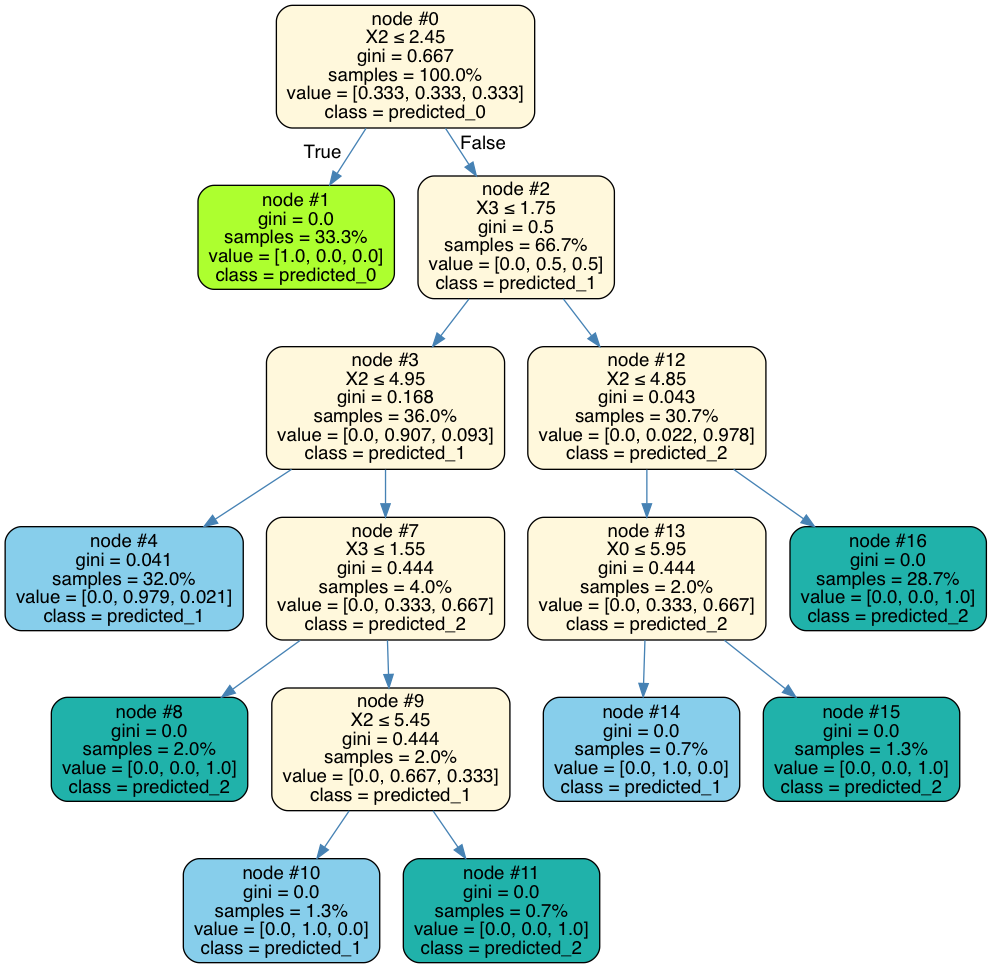Surrogate decision tree

You can use this output to determine the decision boundaries that will be very similar to those in the initial black box model and help to explain why predictions are made given the input feature set.

#### LIME - Local

The method above gives a good explanation for the model from a top-down perspective. For a local explanation using surrogate models, you can use the LIME (Local Interpretable Model-agnostic Explanation) method proposed in a paper by Ribeiro, Singh, Guestrin.

LIME works in a process described the following steps:

1. Choosing an input datapoint for which it will try to explain.
2. Generating additional input data around this datapoint using the distributions from input feature space. The black box algorithm is then applied to this new input data and the results stored.
3. Weight the new samples according to their proximity to the instance of interest.
4. Train a weighted, interpretable model on the dataset with the variations.
5. Explain the prediction by interpreting the local model.

This means that the surrogate model that LIME builds, only needs to accurately reflect the black box model near the point of interest, and hence is free of the sometimes heavy restriction of being an accurate global surrogate.

In the following code, I use an implementation of LIME in the Skater package on the Iris dataset.

```    from skater.core.local_interpretation.lime.lime_tabular import LimeTabularExplainer

exp = LimeTabularExplainer(iris_X.values, feature_names=list(iris_X.columns),
discretize_continuous=True,
class_names=[0, 1, 2])

doc_num = 50
print('Actual Label:', iris_y.iloc[doc_num].values)
print('Predicted Label:', xgb_clf.predict(iris_X.iloc[doc_num]))
exp.explain_instance(iris_X.iloc[doc_num], xgb_clf.predict_proba).show_in_notebook()
```

This prints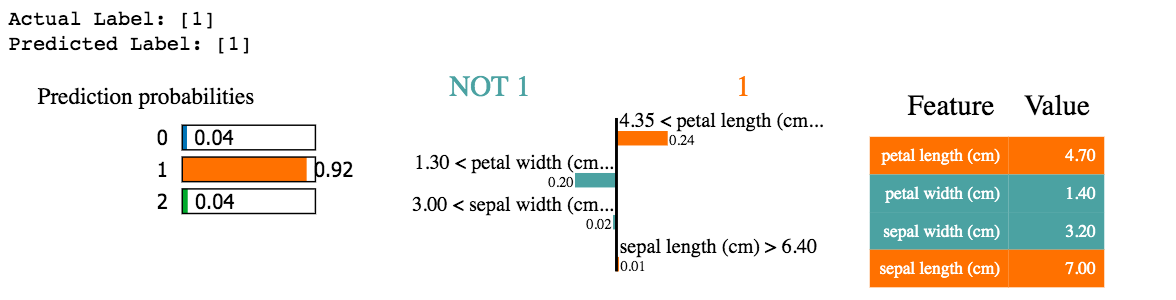Local interpretability

This output shows us the petal length being 4.35 is one of the largest contributors to predicting this datapoint with a value of 1. You can also see which features and their values suggest alternative classes.

### Conclusion

In this post I have shown a few methods you can use to begin to humanise the data science process and explain simply to stakeholders why complex, hard-to-interpet models make the decisions they do. These techniques can help to bridge the gap between simple, business-focused problem solving and mathematically advanced computation techniques, and allow you to explain or validate fundamental connections of natural phenomena when represented by data.